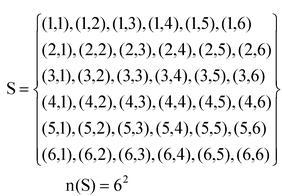# Mind Map

## Probability of occurrence of an event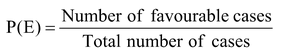Complementary event : Let E be an event and (not E) be an event which occurs only when E does not occur.

The event (not E) is called the complementary event of E.

Clearly, P(E) + P(not E) = 1.

∴P(E) = 1 – P(not E).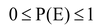Sum of the probabilities of all the outcomes of random experiment is 1

Different Approaches: There are following three types of approaches to theory of probability :

1. Experimental approach, empirical approach or observed required approach.
2. Classical approach.
3. Axiomatic approach.

Some special sample spaces

1. A coin is tossed once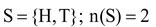2. A coin is tossed twice or

Two coins are tossed simultaneously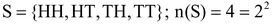1. A coin is tossed three times or

Three coins are tossed simultaneously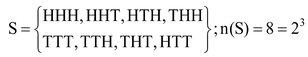Some special sample spaces

1. A die is thrown once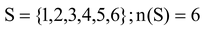2. Two dice are thrown together or

A die is thrown twice